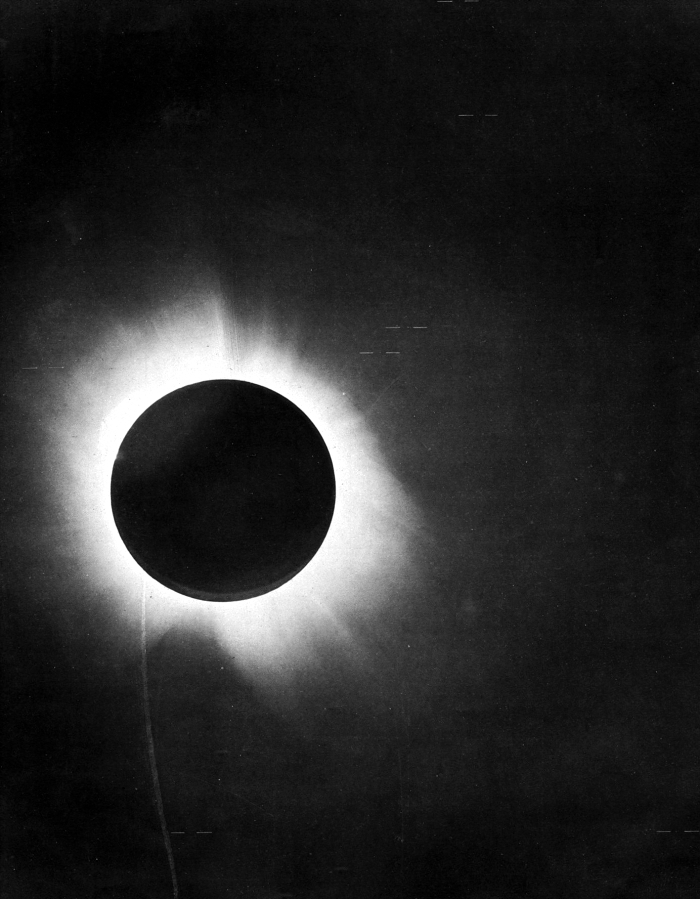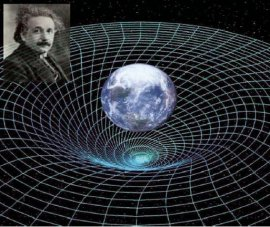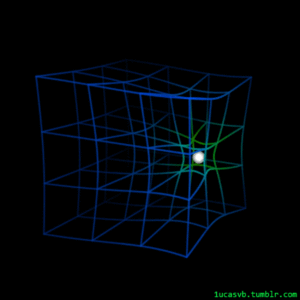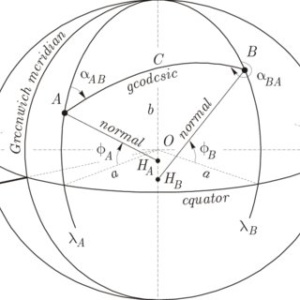# Einstein’s Triumph – This Day 100 Years Ago!A photo of the total solar eclipse of May 29, 1919, from expeditions spearheaded by Sir Arthur Eddington. Scientific observations made during this eclipse proved Einstein’s prediction of the bending of light around the sun. Notice the tick marks around stars near the eclipsed sun. It was the precise measurement of the positions of these stars with respect to the edge of the sun that proved Einstein’s theory. Image credit Wikimedia Commons.

Every year on the 29th of May, the anniversary of the famous 1919 Total Solar Eclipse, we celebrate Einstein’s Triumph, the experiment that provided incontrovertible evidence for the veracity of his theory on Gravity, the General Theory of Relativity.

Up until the turn of the 20th century, the natural universe was governed by Newton’s Laws; the study of Physics was known as “Natural Philosophy” and the compendium of the laws of nature and the mathematics governing them and the universe as we understood it was published in Newton’s famous 1687 work “Philosophiæ Naturalis Principia Mathematica“; translated from the Latin, it reads: “Mathematical Principles of Natural Philosophy“. Newton’s singular genius, concurrent with Leibniz in Germany, gave us Calculus without which the mathematics of the day prior could only approximate what was observed in nature. Along with Calculus, Principia also included Newton’s 3 laws of motion and his Law of Universal Gravitation. For over 200 years, these principles guided and under-girded our understanding of the laws of nature and the ongoing development and expansion of those laws.

A watershed verification of Einstein’s theory on Gravity, his General Theory of Relativity, is what is celebrated today, the Centenary of this measurement, the first of many to test the theory’s veracity. From expeditions spearheaded by Sir Arthur Eddington, scientific observations made during the total solar eclipse of 29 May, 1919, proved Einstein’s prediction of the bending of light in the vicinity of the sun. It was the precise measurement of positions of test stars in the vicinity of the sun both during the eclipse and at another time that provided evidence for the veracity of Einstein’s theory and is what is celebrated on this date.

### Special RelativityAt the turn of the 19th century, working in a Swiss Patent office and fascinated with time, Albert Einstein published in 1905 his seminal work entitled “On the Electrodynamics of Moving Bodies” or more colloquially the “Special Theory of Relativity“, his thesis on the laws of motion governing bodies that are traveling at a significant fraction of the speed of light in an inertial or non-accelerating frame of reference.

The singular foundation of this theory, one that derives from Einstein’s fascination with time, is the invariance of the speed of light. Einstein tackled the seeming contradiction that, as a physical object approaches the speed of light, the relative velocities of light -and the object- don’t add; that is, the measured velocity of light, if its source is located in the frame of reference of the object in motion, is the same as if the object was stationary, in other words, the speed of light is invariant.

Einstein theorized, quite correctly, that the solution to this seeming logical conundrum is quite simply that time slows down for the frame of reference of the object as that object approaches the speed of light. Since velocity is the change in position with time, time has to be the parameter in this equation that changes. Other physical aspects of the moving object change relative to the observer in accordance with the change in time, such as its mass and physical dimension along the direction of motion. These changes are known as the “Lorentz Transformations“, named in honor of the Dutch Physicist and Mathematician Hendrik Lorentz who first derived them as a group in 3 dimensions and which Einstein subsequently incorporated as a fundamental aspect of his Special Theory.

### General Relativity

Newton was quite satisfied with what he provided as an explanation for the behavior of moving objects, not the least of which objects that were moving under the influence of gravity. The paradigm change between Newton’s ideas and Einstein’s, in short, was that Newton accurately described how objects moved while Einstein, in addition to how they moved, described why they moved as they did. In Newton’s “Clockwork Universe“, everything moved according to a fixed set of laws, his 3 laws of motion and his Universal Law of Gravitation. Given enough time, according to Newton, every outcome, ever, could be explained, deduced or, one could argue, predicted; the Universe was fixed, is set in motion and could be reduced to a combination of all that is contained in Principia. As a side note, this thinking, as with Relativity, both Special and General, is completely incompatible with modern Quantum Theory where outcomes are measured and evaluated based on probabilities and very little is known with certainty.

In Newton’s universe, gravity was simply a mutually attractive force, in the simplest case, between two objects, whose magnitude is the product of their masses and the inverse square of the distance separating them. Newton’s gravity law accurately described the motion of objects under the influence of this mysterious force, so much so, that it is used today to compute and predict orbits, trajectories and every aspect of an object’s motion or the integrated motion of a system of objects. Because Newton’s Law of Gravity only describes the behavior of an object under the influence of gravity and not the underlying cause, there are limitations to his law, a notable exception and one known to him, is a peculiarity with the orbit of Mercury and one only -and accurately- described by Einstein’s General Theory of Relativity.

As to the “Why” of gravity, Einstein’s GTR predicts that any object will mutually follow the “curvature of space” produced by each object with a magnitude proportional to each of their masses. This revolutionary idea would not have been possible during Newton’s time and the prevailing provincial attitudes of the day.

The experiment we celebrate today established with scientific certainty, the notion that space is “curved” and that Time is an inseparable aspect to it, hence the term “Space-Time“.

Einstein developed, from-scratch, a derivation of the Lorentz transforms in his 1905 paper, his Special Theory of Relativity. Hermann Minkowski pointed out in 1908 that Einstein’s mathematics were equivalent to the claim that we exist in a (3+1)D manifold (cover), the foundations of “Space-Time“, time being the 4th dimension. It needs to be pointed out without ambiguity that Relativity is completely incompatible with Newtonian gravity – it would be tantamount to comparing apples with bicycles or stones with books, not even apples with oranges since they’re not even in the same genre – one is a fiction, the other, reality.

Attempts to understand special relativity in non-inertial (accelerated frames such as a moving elevator) eventually led to the insight that you could model gravity as a curved manifold. The Lorentz Transforms and the ideas developed in the Special Theory paved the way for Einstein to write his General Theory in 1915.

In 1920 Einstein commented that a thought occurred to him while writing his General Theory that he called “the happiest thought of my life”:

The gravitational field has only a relative existence… Because for an observer freely falling from the roof of a house – at least in his immediate surroundings – there exists no gravitational field.

Lets step back and take a quick look at Special Relativity for a moment, specifically the Lorentz Transforms. Remember the seeming logical contradiction that as an object approaches the speed of light, the speed of light remains invariant if that speed were measured by an observer at any location, i.e., the invariance of the speed of light? If gravity were simply a mutually attractive force, as suggested by Newton, then why do gravitational effects have an immediate effect? This question goes to Einstein’s quote above.

Take for example, the tides as the moon orbits the earth or the change in a satellite’s orbit or the trajectory of a space probe as it nears a planet, the list is endless, just use your imagination. Remember the requirement that as time slows down, that mass increases and the object’s dimension in the direction of motion shrinks, all as you accelerate closer and closer to the speed of light? A corollary to this invariance of the speed of light, that it is always the same regardless of the speed of the source and the speed of the observer, is that no information, signal or object can travel at speeds greater than this, that light is the Universal Speed limit.

1. All other theories must be made compatible with the two postulates of special relativity:
the laws of physics have the same form in the coordinate systems of all observers moving by constant speeds in a constant direction (inertial frames);
2. Constancy of the speed of light: the speed of light is constant, C, regardless of the speed of the source and the speed of the observer;
3. no information, signal or object can travel at speeds greater than this, that light is the Universal Speed limit.A two-dimensional depiction of the local space in the vicinity of the Earth

This constraint of Special Relativity is fundamentally inconsistent with Newton’s notion of Gravity, that it is merely a mutually attractive force. That we observe an instantaneous change in the behavior of any object in a gravitational system, regardless of the distances involved, suggests an infinite speed of transmission for this change, impossible if we accept the third constraint of Special Relativity.

Considering Einstein’s quote above, the only solution therefore is that we accept the notion of a curved physical “Space”, where objects of the system are moving along a curved space whose properties are defined by the masses of the objects and the energies involved. Those objects are then moving in three dimensions, propagating through a fourth dimension of time; hence we have the combined notion of “Space-Time”.Many models and illustrations have been used to depict this idea, an abstraction really, in two dimensions as depressions in a fabric or sheet of some sort, the depth of which is proportional to the mass of the object; i.e., the more massive the object the greater the depression. While this works to illustrate the idea in two dimensions, the reality is quite different.

We live a universe of three physical dimensions propagating through time as the forth dimension. Thus, if we accept this model, reality would require an infinite number of two-dimensional planes centered on an object to depict every such object in a gravitational system where each in the system has the “local space” curved.

While we’re considering our multi-dimensional reality, it is instructive to point out that dimension N is simply the infinite projection of dimension (N – 1); thus 3 dimensions are simply the infinite number of 2 dimensional possibilities. This construct helps us imagine our world in 3 dimensions propagating through time as the fourth dimension. In considering the rapidly evolving models, theories and possibilities of the physical universe in our attempt to understand it in its totality, using such notions as “String Theory”, this exercise should prove helpful and instructive.A simple case of a Geodesic. Note that if we are constrained to travel along the surface, then the path C is the shortest path between A and B. If we travel through the surface, a straight line between A and B, then that would be the shortest distance.

In our three-dimensional reality, the shortest distance between two points is a straight line; many of us have heard this since childhood and it makes perfect logical sense, right? For an infinitely flat plane, then yes, it’s true but not if you consider that we don’t live on an infinitely flat plain. We live in a universe whose very reality is described not as a flat plane but as curved in three dimensions, propagating through time as a forth dimension.

If we consider a Geodesic, a concept in advanced mathematics, that for those who live on a curved surface, as we do, physically on the earth, a spheroidal body that is orbiting in the vicinity of another spheroidal body with considerable mass, the sun, that it then travels along the curved space described by the sun’s mass, then no, the shortest distance between two points is not a straight line. Considering the simplest case of this model, that we are constrained to travel along the surface of the earth, that this is not the shortest distance. If we consider the shortest distance then, for this model, we would have to burrow through the earth if we wanted to travel along the shortest possible path. This model is instructive and can be expanded and extrapolated to describe the curvature of space and the reality of the universe in which we live as a whole.

On four consecutive Thursdays in November of 1915, Einsten presented his General Theory of Relativity to the Prussian Academy of Sciences. In his final lecture, delivered on 25 November, 1915, he presented the following equation to those gathered:

Rμν – ½ Rgμν = 8 π G Tμν

This first iteration of an expression, which came to be known as Einstein’s Field Equations, is written in compact Tensor form. Tensors allow for physical systems to be described in a coordinate independent manner, that is without regard to a certain physical geometry (planar, spherical, cylindrical, etc). This expression describes the gravitational-inertial field, the interplay between the curvature of Space-Time and distances along the curve formed on the Space-Time “fabric” by any object (with mass), expressed on the left side of the equation and the nature of the object itself, the source of the curvature, on the right side.

Now, with all this as a backdrop, we return to our celebration. How did observing the famous eclipse of 29 May, 1919 play a watershed role in our understanding of the Universe and the application of Einstein’s General Theory of Relativity a significant contribution to that understanding?

Take another look at our Geodesic. If the pathways leading to the test stars are curved, then the observation, that the apparent positions of the test stars change in the vicinity of the sun compared to observations with the sun absent, would confirm Einstein’s theory. Well, to within the precision afforded by the equipment of the day, the apparent change in the stars’ position agreed with that predicted by General Relativity! Why did this confirm General Relativity? The light path from the test stars must follow the curvature of space; that path in the vicinity of the sun changes according to the sun’s mass and how that mass warps the local space and thus, the apparent position of the test stars will change.

Why observe during an Eclipse?

Combined with atmospheric scattering during the day, the sun’s brilliance prevents observations of the stars during the day. During a Total Eclipse of the Sun, the sun is briefly covered (eclipsed) thus allowing for such an observation to be conducted.

Total Page Visits: 5908 - Today Page Visits: 1

## 2 thoughts on “Einstein’s Triumph – This Day 100 Years Ago!”

This site uses Akismet to reduce spam. Learn how your comment data is processed.